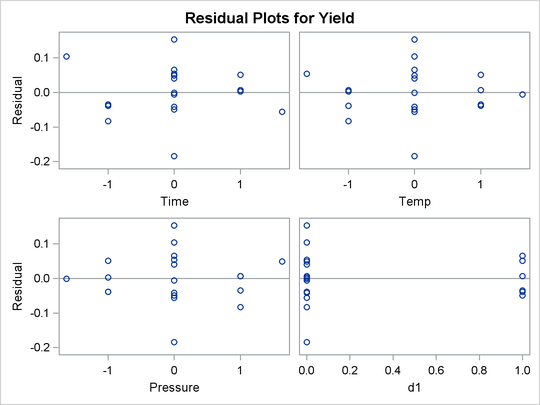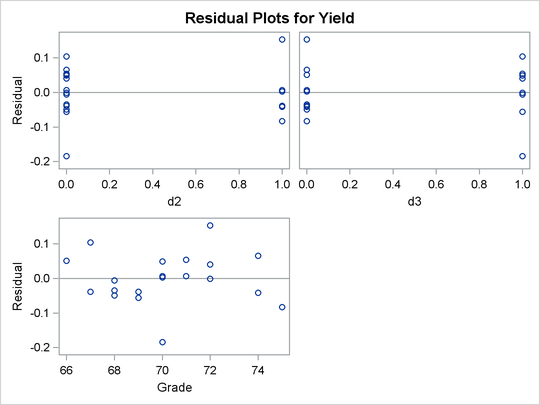### Example 81.2 Response Surface Analysis with Covariates

One way of viewing covariates is as extra sources of variation in the dependent variable that can mask the variation due to primary factors. This example demonstrates the use of the COVAR= option in PROC RSREG to fit a response surface model to the dependent variables corrected for the covariates.

You have a chemical process with a yield that you hypothesize to be dependent on three factors: reaction time, reaction temperature, and reaction pressure. You perform an experiment to measure this dependence. You are willing to include up to 20 runs in your experiment, but you can perform no more than 8 runs on the same day, so the design for the experiment is composed of three blocks. Additionally, you know that the grade of raw material for the reaction has a significant impact on the yield. You have no control over this, but you keep track of it. The following statements create a SAS data set containing the results of the experiment:

```data Experiment;
input Day Grade Time Temp Pressure Yield;
datalines;
1 67      -1     -1     -1         32.98
1 68      -1      1      1         47.04
1 70       1     -1      1         67.11
1 66       1      1     -1         26.94
1 74       0      0      0        103.22
1 68       0      0      0         42.94
2 75      -1     -1      1        122.93
2 69      -1      1     -1         62.97
2 70       1     -1     -1         72.96
2 71       1      1      1         94.93
2 72       0      0      0         93.11
2 74       0      0      0        112.97
3 69       1.633  0      0         78.88
3 67      -1.633  0      0         52.53
3 68       0      1.633  0         68.96
3 71       0     -1.633  0         92.56
3 70       0      0      1.633     88.99
3 72       0      0     -1.633    102.50
3 70       0      0      0         82.84
3 72       0      0      0        103.12
;
```

Your first analysis neglects to take the covariates into account. The following statements use PROC RSREG to fit a response surface to the observed yield, but note that `Day` and `Grade` are omitted:

```proc rsreg data=Experiment;
model Yield = Time Temp Pressure;
run;
```

The ANOVA results shown in Output 81.2.1 indicate that no process variable effects are significantly larger than the background noise.

Output 81.2.1: Analysis of Variance Ignoring Covariates

The RSREG Procedure

Regression DF Type I Sum of Squares R-Square F Value Pr > F
Linear 3 1880.842426 0.1353 0.67 0.5915
Quadratic 3 2370.438681 0.1706 0.84 0.5023
Crossproduct 3 241.873250 0.0174 0.09 0.9663
Total Model 9 4493.154356 0.3233 0.53 0.8226

Residual DF Sum of Squares Mean Square
Total Error 10 9405.129724 940.512972

However, when the yields are adjusted for covariate effects of day and grade of raw material, very strong process variable effects are revealed. The following statements produce the ANOVA results in Output 81.2.2. Note that in order to include the effects of the classification factor `Day` as covariates, you need to create dummy variables indicating each day separately.

```data Experiment;
set Experiment;
d1 = (Day = 1);
d2 = (Day = 2);
d3 = (Day = 3);
```
```ods graphics on;
proc rsreg data=Experiment plots=all;
model Yield = d1-d3 Grade Time Temp Pressure / covar=4;
run;
ods graphics off;
```

The results show very strong effects due to both the covariates and the process variables.

Output 81.2.2: Analysis of Variance Including Covariates

The RSREG Procedure

Regression DF Type I Sum of Squares R-Square F Value Pr > F
Covariates 3 13695 0.9854 316957 <.0001
Linear 3 156.524497 0.0113 3622.53 <.0001
Quadratic 3 22.989775 0.0017 532.06 <.0001
Crossproduct 3 23.403614 0.0017 541.64 <.0001
Total Model 12 13898 1.0000 80413.2 <.0001

Residual DF Sum of Squares Mean Square
Total Error 7 0.100820 0.014403

The number of observations in the data set might be too small for the diagnostic plots in Output 81.2.3 to dependably identify problems; however, some outliers are indicated. The residual plots in Output 81.2.4 do not display any obvious structure.

Output 81.2.3: Fit DiagnosticsOutput 81.2.4: Residual Plots